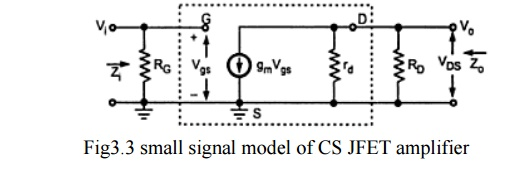Home | | Electronic Circuits I | Common Source Amplifier With Fixed Bias

# Common Source Amplifier With Fixed Bias

Figure shows Common Source Amplifier With Fixed Bias. The coupling capacitor C1 and C2 which are used to isolate the d.c biasing from the applied ac signal act as short circuits for ac analysis.

Common Source Amplifier With Fixed Bias

Figure shows Common Source Amplifier With Fixed Bias. The coupling capacitor C1 and C2 which are used to isolate the d.c biasing from the applied ac signal act as short circuits for ac analysis.The following figure shows the low frequency equivalent model for Common Source Amplifier With Fixed Bias. It is drawn by replacing

·        All capacitors and d.c supply voltages with short circuit

·        JFET with its low frequency a.c Equivalent circuitInput Impedance Zi

Zi = RG

Output Impedance ZoIt is the impedance measured looking from the output side with input voltage Vi equal to Zero. As Vi=0,Vgs =0 and hence gmVgs =0 . And it allows current source to be replaced by an open circuit.

So,If the resistance rd is sufficiently large compared to RD, thenStudy Material, Lecturing Notes, Assignment, Reference, Wiki description explanation, brief detail
Electronic Circuits : JFET and MOSFET Amplifiers : Common Source Amplifier With Fixed Bias |